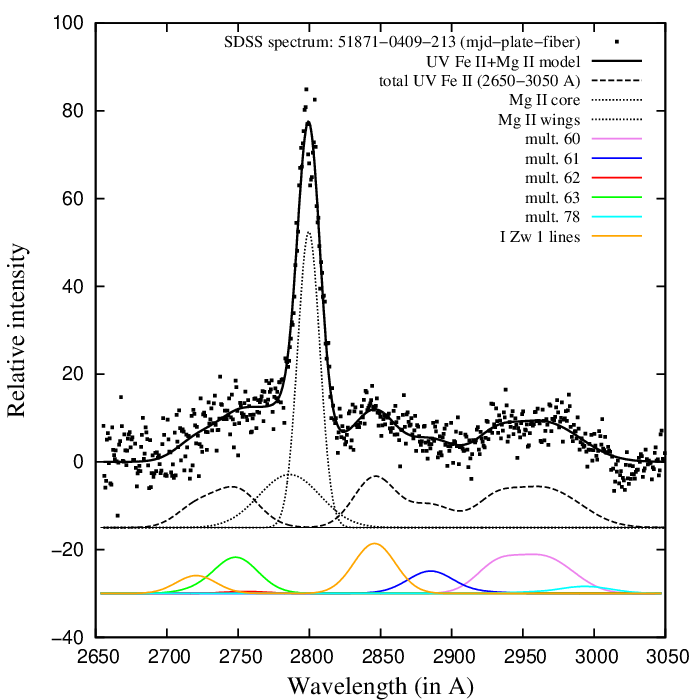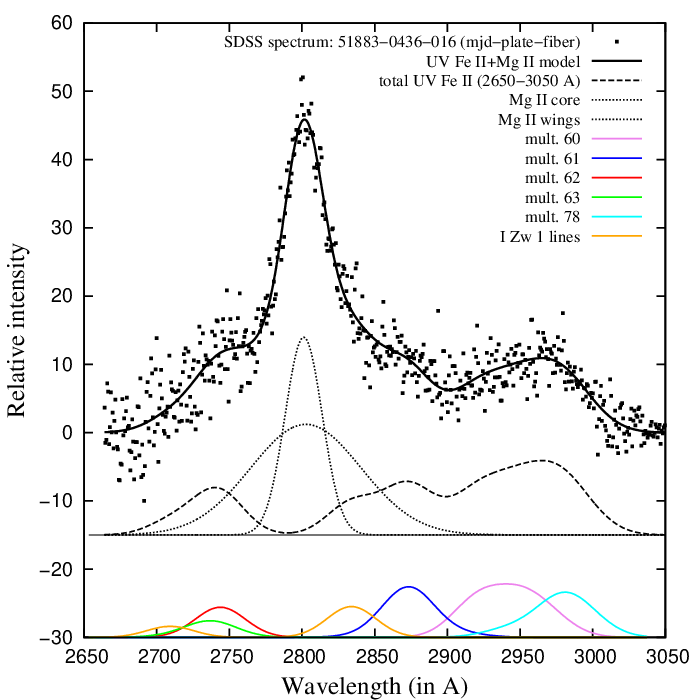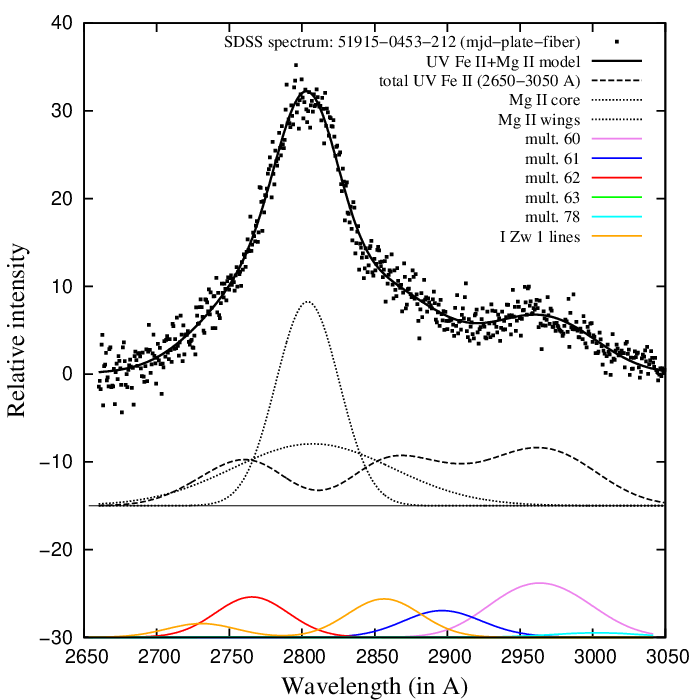# Modelling the UV Fe II lines in the 2650-3050 A range - template download

Here you may download the UV Fe II template in 2650-3050 A range, presented in Appendix A of paper Popovic et al. 2018. The template consists of the five Fe II multiplets (60, 61, 62, 63 and 78) and additional 'I Zw 1 lines' in ascii form, calculated for different widths and shifts of the UV Fe II lines. The relative intensities of the lines within each multiplet are calculated using formula (1) from Popovic et al. 2018, for temperature T = 10000 K, while relative intensities of the 'I Zw 1 lines' are obtained empirically. The FWHM range of given templates is from 1000 km/s to 10000 km/s (with a step of 200 km/s), and the shift range (relative to laboratory wavelength) is from -2000 km/s to 2000 km/s (with a step of 500 km/s).

The UV Fe II templates for different pairs of shifts and widths are given in 414 folders.

In each folder, there are ASCII files of the five Fe II multiplets (60, 61, 62, 63 and 78) and additional 'I Zw 1 lines' (see the Appendix A in Popovic et al. 2018). They are shown with different colours in figures given in each folder (UVFeII_shift_FWHM.ps), and their sum (the total template) is denoted with black, solid line. The multiplets have arbitrary relative intensities, and their relative intensities are the same in all folders.

In process of fitting of observed spectra, the Fe II multiplets and 'IZw1 lines' intensities should be rescaled (multiplied with different scalars) in order to find the best fit of the UV Fe II lines.

## The examples of the UV Fe II template

Figure 1. UV Fe II parameters: FWHM = 3610 km/s, shift = 610 km/s, relative intensities of the multiplets: I(60) = 6.65, I(61) = 4.01, I(62) = 0.16, I(63) = 4.42, I(78) = 0.53, I('IZw1 lines') =11.39.Figure 2. UV Fe II parameters: FWHM = 4170 km/s, shift = - 630 km/s, relative intensities of the multiplets: I(60) = 5.14, I(61) = 5.69, I(62) = 1.55, I(63) = 1.26, I(78) = 2.09, I('IZw1 lines') =4.50.Figure 3. UV Fe II parameters: FWHM = 6225 km/s, shift = 1745 km/s, relative intensities of the multiplets: I(60) = 3.17, I(61) = 2.23, I(62) = 1.57, I(63) = 0.01, I(78) = 0.15, I('IZw1 lines') =4.38.« Home